VillainHR

## SVG 快速入门

2017-04-17

SVG 全称是 Scalable Vector Graphics，即，矢量图。在 Web 中使用 SVG 可以解决位图放大失真的问题。首先，不要把 SVG 和 CSS，Canvas，HTML 搞混。他们之间并没有你中有我，我中有你的关系。SVG 是通过 XML 的形式写在 HTML 文档中的。

## 如何书写

``````<svg x="0px" y="0px" width="450px" height="100px" viewBox="0 0 450 100">
<rect x="10" y="5" fill="white" stroke="black" width="90" height="90"/>
<circle fill="white" stroke="black" cx="170" cy="50" r="45"/>
<polygon fill="white" stroke="black" points="279,5 294,35 328,40 303,62
309,94 279,79 258,94 254,62 230,39 263,35"/>
<line fill="none" stroke="black" x1="410" y1="95" x2="440" y2="6"/>
<line fill="none" stroke="black" x1="360" y1="6" x2="360" y2="95"/>
</svg>
``````

## 基本概念

• viewport: 物理窗口
• viewbox: 实物窗口（算了，下面解释）
• preserveAspectRatio: 保留横纵比

### viewbox

``````<svg width="500" height="200" viewBox="0 0 50 20" >
<rect x="20" y="10" width="10" height="5"
style="stroke: #000000; fill:none;"/>
</svg>
``````
• viewport 为 [0,0] 到 [500,200]
• viewbox 为 [0,0] 到 [50,20]

``````<rect x="20" y="10" width="10" height="5"
style="stroke: #000000; fill:none;"/>
``````

### preserveAspectRatio

``````<align> [<meetOrSlice>]
``````
• align: 定义 viewport 和 viewbox 的对齐方式，分为 x,y 轴两个方向。X 轴方向有三种方式：左边重合（xMin），x 轴中点重合（xMid），右边重合（xMax）。同理，Y 轴也有 顶边重合（YMin），y 轴中点重合（YMid），底边边重合（YMax）
• meetOrSlice: 主要就是定义该 SVG 是内嵌，还是裁剪或是 none（听天有命）。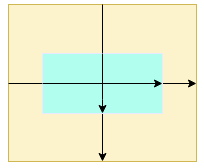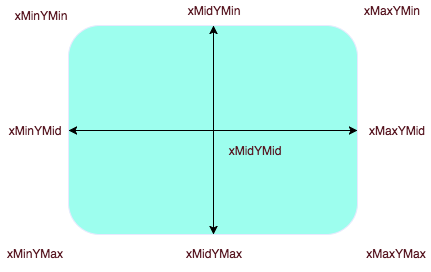vb_h * rat_y = vp_h; 或者 vb_w * rat_x = vp_w;

``````<svg width="400" height="200" viewBox="0 0 200 200" preserveAspectRatio="xMinYMin slice" style="border:1px solid #cd0000;">
<rect x="10" y="10" width="150" height="150" fill="#cd0000"/>
</svg>
``````

• rat_x = 400/200 = 2
• rat_y = 200/200 = 1

• meet(默认值): 本意是让 svg 尽可能的显示在 viewport 里，即，会在 rat_x 和 rat_y 中选择最小的值作为缩放标准。
• slice: 本意是让 svg 完全铺满 viewport，即，会在 rat_x 和 rat_y 中选择最大的值作为缩放标准。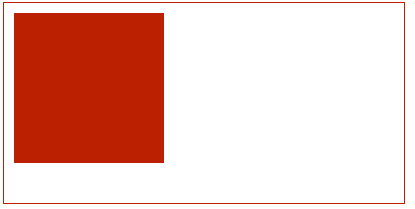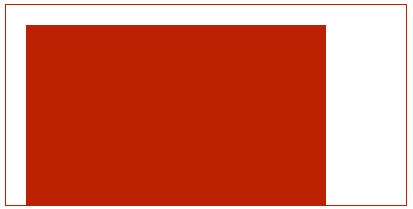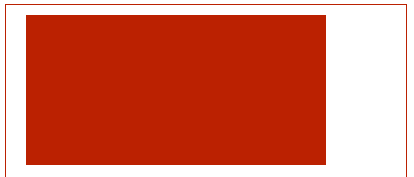### 响应式 SVG

``````<svg viewBox="0 0 218.8 87.1">
<g fill="none" stroke="#000">
<path d="M7.3 75L25.9 6.8s58.4-6.4 33.5 13-41.1 32.8-11.2 30.8h15.9v5.5s42.6
18.8 0 20.6" />
<path d="M133.1 58.2s12.7-69.2 24.4-47.5c0 0 4.1 8.6 9.5.9 0 0 5-10 10.4.9 0
0 12.2 32.6 13.6 43 0 0 39.8 5.4 15.8 15.4-13.2 5.5-53.8
13.1-77.4 5.9.1 0-51.9-15.4 3.7-18.6z" />
</g>
</svg>
``````

• rect
• circle
• ellipse
• line
• polyline
• polygon

## 分组和 Path

``````<g fill="none" stroke="#000">
<path d="M7.3 75L25.9 6.8s58.4-6.4 33.5 13-41.1 32.8-11.2 30.8h15.9v5.5s42.6
18.8 0 20.6" />
<path d="M133.1 58.2s12.7-69.2 24.4-47.5c0 0 4.1 8.6 9.5.9 0 0 5-10 10.4.9 0
0 12.2 32.6 13.6 43 0 0 39.8 5.4 15.8 15.4-13.2 5.5-53.8
13.1-77.4 5.9.1 0-51.9-15.4 3.7-18.6z" />
</g>
``````

### Path

Path 在 SVG 中的地位应该是比较高的，实际上，利用 Path 这个一个标签可以画出任意的图形。path 中 `d`(data) 属性是用来定义相关线条数据，通常是以 `M/m` 为起始，代表的就是 `move to` 的意思。在 path 中，一共可以定义 10 种不同的图形。例如 `M/m``L/l`。 大家可以注意，每种标识符有两种书写方式，即，大小写。

• 大写: 参照的是绝对坐标，即，SVG 的右上角
• 小写: 参照的相对坐标，即，前一个点的坐标。

### 线型

#### M/m

``````<path d="M10 10"/>
``````

#### L/l

``````L x y (or l dx dy)
``````

#### H/h

``````H x (or h dx)
``````

#### V/v

``````V y (or v dy)
``````

``````<path d="M10 10 H 90 V 90 H 10 L 10 10"/>
``````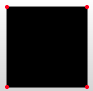#### Z/z

``````Z (or z)
``````

``````<path d="M10 10 H 90 V 90 H 10 L 10 10"/>
// 使用 Z
<path d="M10 10 H 90 V 90 H 10 Z" fill="transparent" stroke="black"/>
``````

### 曲线

#### C/c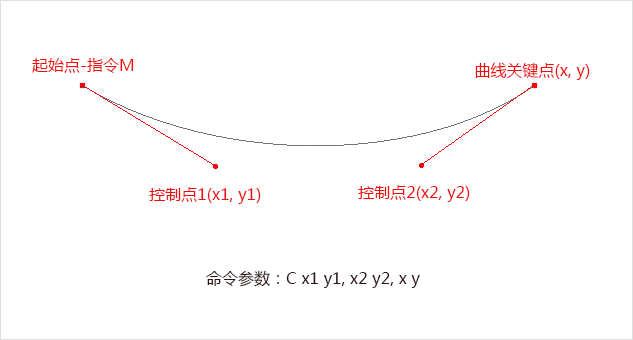``````C x1 y1, x2 y2, x y (or c dx1 dy1, dx2 dy2, dx dy)
``````

``````<path d="M10 10 C 20 20, 40 20, 50 10" stroke="black" fill="transparent"/>
``````

#### S/s

``````S x2 y2, x y (or s dx2 dy2, dx dy)
``````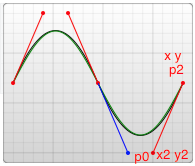``````<path d="M10 80 C 40 10, 65 10, 95 80 S 150 150, 180 80" stroke="black" fill="transparent"/>
``````

#### Q/q``````Q x1 y1, x y (or q dx1 dy1, dx dy)
``````

``````<path d="M10 80 Q 95 10 180 80" stroke="black" fill="transparent"/>
``````

#### T/t

``````T x y (or t dx dy)
``````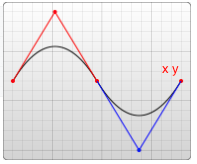### 弧线

#### A/a

``````A rx ry x-axis-rotation large-arc-flag sweep-flag x y
a rx ry x-axis-rotation large-arc-flag sweep-flag dx dy
``````

• rx,ry: 代表的就是长轴短轴，没得说。
• x,y: 代表的是弧长的结束点。开始点就是上一个命令的终点。
• x-axis-rotation: x 轴的旋转角度。顺时针为正
• large-arc-flag[0,1]: 表示取大弧还是小弧。因为两点之间的弧长有两部分。
• sweep-flag[0,1]: 取顺时针的弧，还是逆时针的弧长。参考点是以起始点开始的。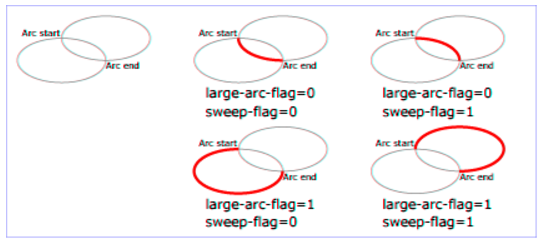### 分组

SVG 中的分组你可以理解为 PS 中的图层，一块图层里面通常只会放一下高内聚的图形，这样既方便移动又方便做动画。SVG 中的分组标签就是 `g`，使用 `g` 标签包裹的所有子元素都认同为一组。

`````` <g>
<circle cx="20" cy="20" r="20" fill="green" />
<circle cx="70" cy="70" r="20" fill="purple" />
</g>
<g>
<rect x="110" y="110" height="30" width="30" fill="blue" />
<rect x="160" y="160" height="30" width="30" fill="red" />
</g>
``````

#### 分组动画

``````<g transform="translate(...) scale(...) rotate(...) translate(...) rotate(...)">
...
</g>
``````

``````<g transform="translate(...) scale(...) rotate(...) translate(...) rotate(...)">
...
</g>

// Being Equivalent to this:
<g transform="translate(...)">
<g transform="scale(...)">
<g transform="rotate(...)">
<g transform="translate(...)">
<g transform="rotate(...)">
...
</g>
</g>
</g>
</g>
</g>
``````